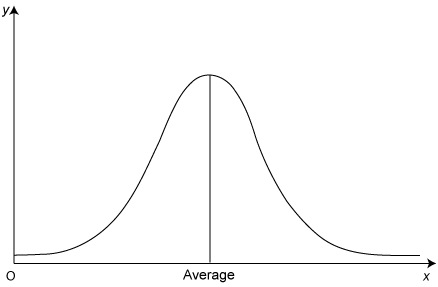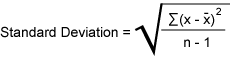## Analysis Intermediate: Evaluating Risk# Standard Deviation Reflects Investment Volatility

Analysts can use a statistical concept called standard deviation to measure an investment’s volatility, the degree to which a security’s price moves up and down. The higher an investment’s volatility, the higher its investment risk.

### Things To Know

• Standard deviation measures how much a security’s prices vary from its expected average.
• Most of a security’s price variations fall within one standard deviation of its mean.

## What is standard deviation?

Over time, as a security’s price changes, the prices tend to distribute themselves around an average price. Most of the time, the security’s price will remain close to its average. However, occasionally the price moves further away from the average. Statisticians refer to this type of scattering as a normal distribution. Standard deviation is a statistical measure of how much a security’s prices vary from this expected average. A high standard deviation means the price change is very volatile, while a low standard deviation means the price is not very volatile.

A normal distribution curve looks like this:## What the curve represents

The x-axis represents the price range of the security, and the y-axis represents the number of times the security traded at a particular price. The vertical line that divides the curve is the average price. When the curve is steep, a security’s standard deviation is small. A flat curve represents a large standard deviation. The higher the standard deviation, the higher the security’s volatility risk. Statisticians calculate the standard deviation using the following formula:Almost 70 percent of a security’s price variations will fall within one standard deviation of its mean. To get an accurate understanding of a security’s true volatility risk, you will need to compare the deviation of one security with that of another.